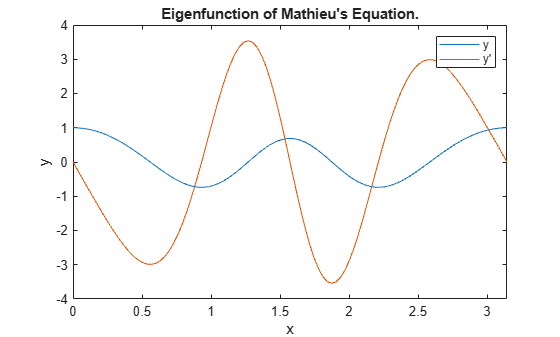# Solve BVP with Unknown Parameter

This example shows how to use `bvp4c` to solve a boundary value problem with an unknown parameter.

Mathieu's equation is defined on the interval $\left[0,\pi \right]$ by

${{\mathit{y}}^{\prime }}^{\prime }+\left(\lambda -2\mathit{q}\text{\hspace{0.17em}}\mathrm{cos}\left(2\mathit{x}\right)\right)\mathit{y}=0$.

When the parameter $\mathit{q}=5$, the boundary conditions are

${\mathit{y}}^{\prime }\left(0\right)=0$,

${\mathit{y}}^{\prime }\left(\pi \right)=0$.

However, this only determines $\mathit{y}\left(\mathit{x}\right)$ up to a constant multiple, so a third condition is required to specify a particular solution,

$\mathit{y}\left(0\right)=1$.

To solve this system of equations in MATLAB, you need to code the equations, boundary conditions, and initial guess before calling the boundary value problem solver `bvp4c`. You can either include the required functions as local functions at the end of a file (as done here), or save them as separate, named files in a directory on the MATLAB path.

### Code Equation

Create a function to code the equations. This function should have the signature `dydx = mat4ode(x,y,lambda)`, where:

• `x` is the independent variable.

• `y` is the dependent variable.

• `lambda` is an unknown parameter representing an eigenvalue.

You can write Mathieu's equation as a first-order system using the substitutions ${\mathit{y}}_{1}=\mathit{y}$ and ${\mathit{y}}_{2}={\mathit{y}}^{\prime }$,

${{\mathit{y}}_{1}}^{\prime }={\mathit{y}}_{2}$,

${{\mathit{y}}_{2}}^{\prime }=-\left(\lambda -2\mathit{q}\text{\hspace{0.17em}}\mathrm{cos}\left(2\mathit{x}\right)\right){\mathit{y}}_{1}$.

The corresponding function is then

```function dydx = mat4ode(x,y,lambda) % equation being solved dydx = [y(2) -(lambda - 2*q*cos(2*x))*y(1)]; end ```

Note: All functions are included as local functions at the end of the example.

### Code Boundary Conditions

Now, write a function that returns the residual value of the boundary conditions at the boundary points. This function should have the signature `res = mat4bc(ya,yb,lambda)`, where:

• `ya` is the value of the boundary condition at the beginning of the interval $\left[\mathit{a},\mathit{b}\right]$.

• `yb` is the value of the boundary condition at the end of the interval $\left[\mathit{a},\mathit{b}\right]$.

• `lambda` is an unknown parameter representing an eigenvalue.

This problem has three boundary conditions in the interval $\left[0,\pi \right]$. To calculate the residual values, you need to put the boundary conditions into the form $\mathit{g}\left(\mathit{x},\mathit{y}\right)=0$. In this form the boundary conditions are

${\mathit{y}}^{\prime }\left(0\right)=0$,

${\mathit{y}}^{\prime }\left(\pi \right)=0$,

$\mathit{y}\left(0\right)-1=0$.

The corresponding function is then

```function res = mat4bc(ya,yb,lambda) % boundary conditions res = [ya(2) yb(2) ya(1)-1]; end ```

### Create Initial Guess

Lastly, create an initial guess of the solution. You must provide an initial guess for both solution components ${\mathit{y}}_{1}=\mathit{y}\left(\mathit{x}\right)$ and ${\mathit{y}}_{2}={\mathit{y}}^{\prime }\left(\mathit{x}\right)$, as well as the unknown parameter $\lambda$. Only eigenvalues and eigenfunctions that are close to the initial guesses can be computed. To increase the likelihood that the computed eigenfunction corresponds to the fourth eigenvalue, you should choose an initial guess that has the correct qualitative behavior.

For this problem, a cosine function makes for a good initial guess since it satisfies the three boundary conditions. Code the initial guess for $\mathit{y}$ using a function that returns the guess for ${\mathit{y}}_{1}$ and ${\mathit{y}}_{2}$.

```function yinit = mat4init(x) % initial guess function yinit = [cos(4*x) -4*sin(4*x)]; end ```

Call `bvpinit` using a mesh of 10 points in the interval $\left[0,\pi \right]$, the initial guess function, and a guess of 15 for the value of $\lambda$.

```lambda = 15; solinit = bvpinit(linspace(0,pi,10),@mat4init,lambda);```

### Solve Equation

Call `bvp4c` with the ODE function, boundary condition function, and initial guess.

`sol = bvp4c(@mat4ode, @mat4bc, solinit);`

### Value of Parameter

Print the value of the unknown parameter $\lambda$ found by `bvp4c`. This value is the fourth eigenvalue ($\mathit{q}=5$) of Mathieu's equation.

```fprintf('Fourth eigenvalue is approximately %7.3f.\n',... sol.parameters)```
```Fourth eigenvalue is approximately 17.097. ```

### Plot Solution

Use `deval` to evaluate the solution computed by `bvp4c` at 100 points in the interval $\left[0,\pi \right]$.

```xint = linspace(0,pi); Sxint = deval(sol,xint);```

Plot both solution components. The plot shows the eigenfunction (and its derivative) associated with the fourth eigenvalue ${\lambda }_{4}=17.097$.

```plot(xint,Sxint) axis([0 pi -4 4]) title('Eigenfunction of Mathieu''s Equation.') xlabel('x') ylabel('y') legend('y','y''')```### Local Functions

Listed here are the local helper functions that the BVP solver `bvp4c` calls to calculate the solution. Alternatively, you can save these functions as their own files in a directory on the MATLAB path.

```function dydx = mat4ode(x,y,lambda) % equation being solved q = 5; dydx = [y(2) -(lambda - 2*q*cos(2*x))*y(1)]; end %------------------------------------------- function res = mat4bc(ya,yb,lambda) % boundary conditions res = [ya(2) yb(2) ya(1)-1]; end %------------------------------------------- function yinit = mat4init(x) % initial guess function yinit = [cos(4*x) -4*sin(4*x)]; end %-------------------------------------------```